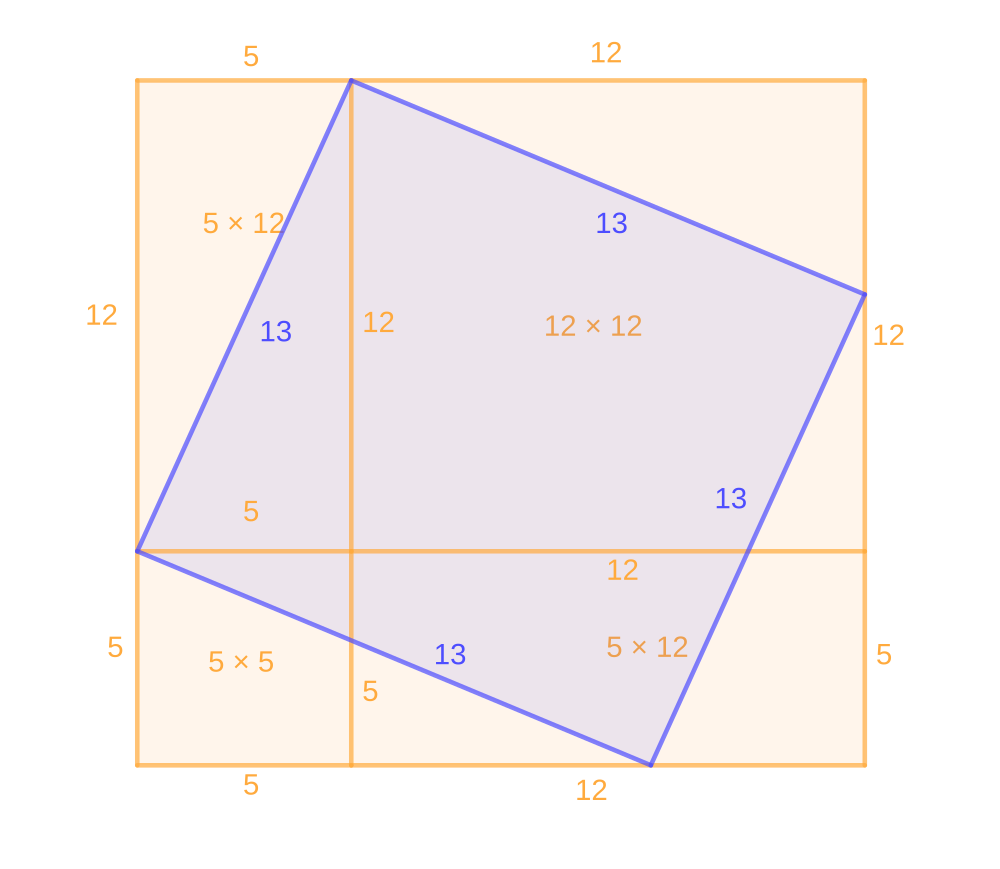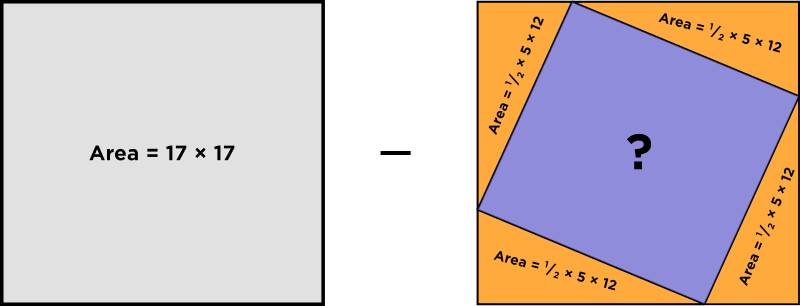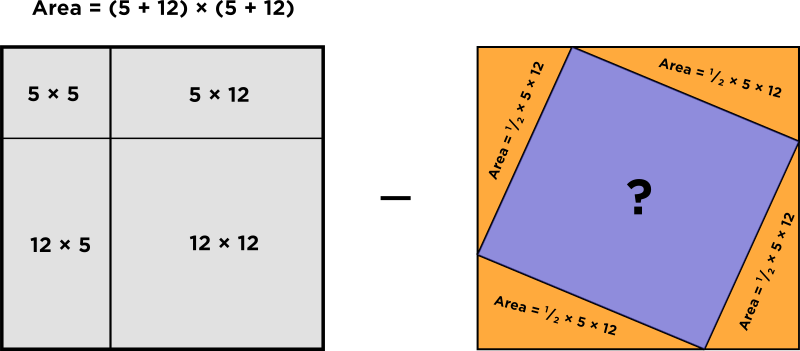# Where did you get the 5² and 12² from?

• [Originally posted in the Discussions forum]

Module 0 Week 1 Day 1 Your Turn

Hi,

I'm glad you asked, because this topic is really nifty. The reason for the $$5^2$$ and $$12^2$$ harks back to the Day 1 Challenge Explanation, when we expanded $$(5 + 12)^2.$$ Let's rewind...

Instead of the right triangle with sides of $$3, 4,$$ and $$5$$ that was used in the Day 1: Challenge, let's use the numbers from our problem, to get right triangles with legs of $$5$$ and $$12.$$We want to get an expression that relates $$5^2$$ and $$12^2,$$ so the trick is to describe the area of the purple square in two ways.

### First way: Use $$17$$ as the side of the large square

$$17^2 - (\text{area of the } 4 \text{ right } \bigtriangleup s)$$

The first way gives us:

\begin{aligned} \text{ Area of purple square } &= 17^2 - 4 × \left( \frac{1}{2} × 5 × 12 \right) \\ &= 17^2 - 120 \\ &= 289 - 120 \\ &= 169 \\ &= 13^2 \\ \end{aligned}### Second way: Use 5 + 12 as the side of the large square, and expand

$$\text{ Area of the purple square } = (5 + 12)^2 - \left( \text{area of the } 4 \text{ right } \bigtriangleup s \right)$$

\begin{aligned} \text{ Area of purple square } + &= (5 + 12)^2 - 4 × \left( \frac{1}{2} × 5 × 12 \right) \\ &= ( 5^2 + 2×5×12 + 12^2 ) - 2×5×12 \\ &= 5^2 + 12^2 + 2×5×12 - 2×5×12 \\ &= 5^2 + 12^2 \\ \end{aligned}These ways both are equivalent to the area of the purple square, so let's set them equal to each other!

$$5^2 + 12^2 = 13^2$$

This illustrates the Pythagorean Theorem, which generalizes to say that

$$(\text{ short leg })^2 + (\text{ long leg })^2 = (\text{ hypotenuse })^2$$

(The legs of a right triangle are the ones that meet at a right angle.)

So, whenever you see a right triangle, you know that you can add the square of the short leg to the square of the longer leg, and that will equal the square of the length of the hypotenuse!

Did that help? I hope so.Let me know!

Happy Learning!